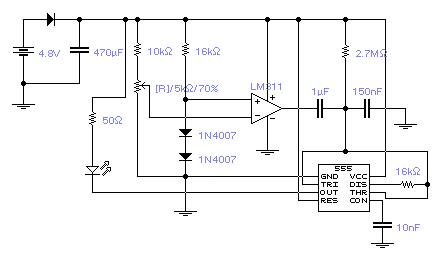Accu Voltage Controller for 4 or 5 NiCd Cells

This Low-Power Battery Voltage Controller for RC Models is flashing with approx. 0.6 Hz, indicating proper function, if the battery voltage is higher than a adjustable reference voltage. If the battery voltage drops below the reference voltage, the LED starts flashing with 5 Hz.
Recommended reference voltage : 4.85 - 4.95 V if 4 NiCd Cells are used

Function :
If Ud is positive at the LM 311, that means battery voltage is below Uref, the output of the LM 311 is floating (Open Collector of LM 311), so the 1u C is not active (T = 2.7M * 150n). If Ud is negative, that means battery voltage exceeds Uref, the output is low (Ground), so the 1u C is active (T = 2.7M * (1u + 150n)).

Power Consumption: 5 - 9 mAh